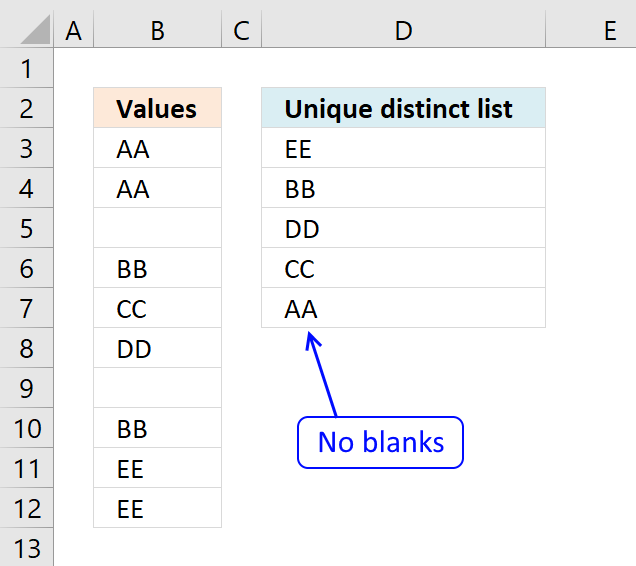Author: Oscar Cronquist Article last updated on December 23, 2018

Question: How do I create a unique distinct list from a list containing several blanks?

Answer: Cell range B3:B12 contains several blank cells. The following formula in cell D3 extracts unique distinct values from cell range B3:B12. Unique distinct values are all values except duplicates.Formula in 32:

=LOOKUP(2, 1/((COUNTIF(\$D\$2:D2, \$B\$3:\$B\$12)=0)*(\$B\$3:\$B\$12<>"")), \$B\$3:\$B\$12)

Copy cell B2 and paste to cells below.

Recommended article

Create a unique distinct alphabetically sorted list

The array formula in cell D3 extracts unique distinct values sorted A to Z, from column B to column D. […]

### Explaining formula in cell D3

#### Step 1 - Check cell range B3:B12 for non-empty cells

If a cell contains a value TRUE is returned. The following line is a logical expression, if cells is not equal to nothing return TRUE.

\$B\$3:\$B\$12<>""

becomes

{"AA";"AA";0;"BB";"CC";"DD";0;"BB";"EE";"EE"}<>""

and returns

{TRUE;TRUE; FALSE;TRUE; TRUE;TRUE; FALSE;TRUE; TRUE;TRUE}

#### Step 2 - Ignore duplicate cells

COUNTIF(\$D\$2:D2, \$B\$3:\$B\$12)=0

becomes

COUNTIF("Unique distinct list",{"AA";"AA";0;"BB";"CC";"DD";0;"BB";"EE";"EE"})=0

becomes

{0;0;0;0;0;0;0;0;0;0}=0

and returns {TRUE; TRUE; TRUE; TRUE; TRUE; TRUE; TRUE; TRUE; TRUE; TRUE}

How to use the COUNTIF function

Counts the number of cells that meet a specific condition.

#### Step 3 - Multiply arrays

(COUNTIF(\$D\$2:D2, \$B\$3:\$B\$12)=0)*(\$B\$3:\$B\$12<>"")

becomes

{TRUE; TRUE; TRUE; TRUE; TRUE; TRUE; TRUE; TRUE; TRUE; TRUE}* {TRUE;TRUE; FALSE;TRUE; TRUE;TRUE; FALSE;TRUE; TRUE;TRUE}

and returns {1;1;0;1;1;1;0;1;1;1}

#### Step 4 - Divide 1 with array

1/((COUNTIF(\$D\$2:D2, \$B\$3:\$B\$12)=0)*(\$B\$3:\$B\$12<>""))

becomes

1/({1;1;0;1;1;1;0;1;1;1})

and returns {1;1;#DIV/0!;1;1;1;#DIV/0!;1;1;1}

#### Step 5 - Find last match in array and return corresponding value

LOOKUP(2, 1/((COUNTIF(\$D\$2:D2, \$B\$3:\$B\$12)=0)*(\$B\$3:\$B\$12<>"")), \$B\$3:\$B\$12)

becomes

LOOKUP(2, {1;1;#DIV/0!;1;1;1;#DIV/0!;1;1;1}, \$B\$3:\$B\$12)

becomes

LOOKUP(2, {1;1;#DIV/0!;1;1;1;#DIV/0!;1;1;1}, {"AA";"AA"; 0;"BB";"CC"; "DD";0;"BB"; "EE";"EE"})

and returns "EE" in cell D3.

How to use the LOOKUP function

Finds a value in a sorted cell range and returns a value on the same row.